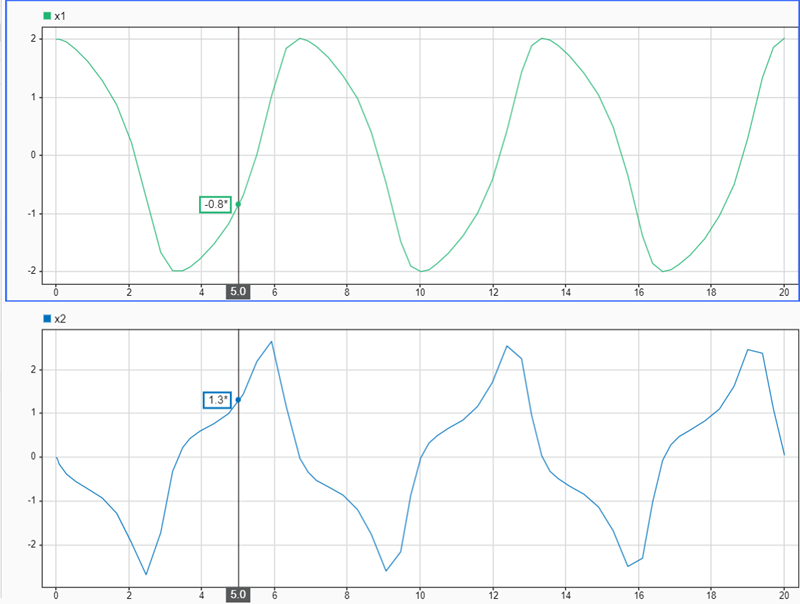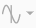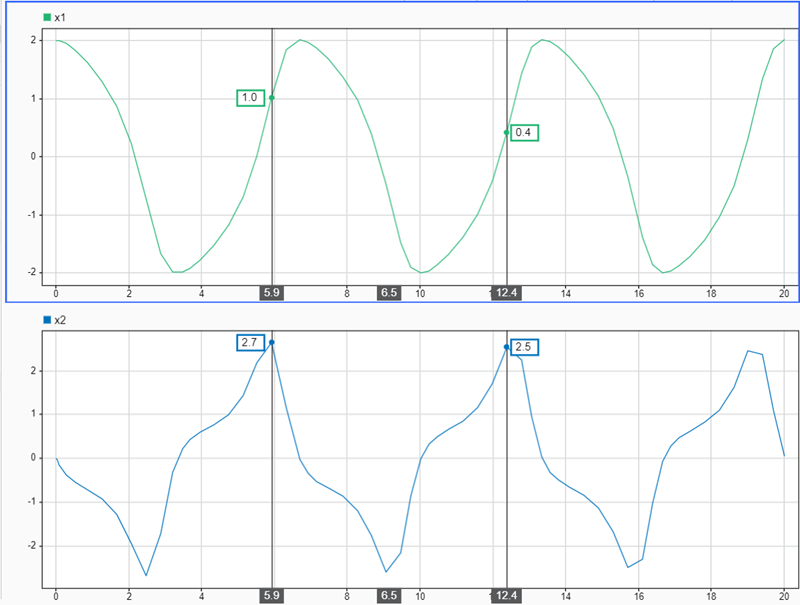Specify active cursor positions in the Simulation Data Inspector

## Syntax

``Simulink.sdi.setCursorPositions(Name,Value)``

## Description

example

````Simulink.sdi.setCursorPositions(Name,Value)` configures the position for active cursors in the Simulation Data Inspector as specified by one or more name-value pair arguments.```

## Examples

collapse all

You can use the Simulation Data Inspector programmatic interface to specify the position of cursors on time plots or sparklines and to access the time that corresponds to the current cursor position.

Open the session file `vdp_mu.mldatx` to add plot data in the Simulation Data Inspector. The session file contains data for a 20-second simulation of a model of the Van Der Pol equation, with signals `x1` and `x2` plotted on separate subplots in a `2`-by-`1` subplot layout.

`open vdp_mu.mldatx;`

Use the `Simulink.sdi.setNumCursors` function to add one cursor to the plot.

`Simulink.sdi.setNumCursors(1);`

Suppose you want to know the signal values `5` seconds into the simulation. Use the `Simulink.sdi.setCursorPositions` function to move the cursor to `t=5s` and read the signal values off from the cursor on each subplot. The asterisk next to the value in the cursor label indicates that the value is interpolated because the simulation did not include a calculation for a simulation time of exactly `5s`.

`Simulink.sdi.setCursorPositions('left',5);`Suppose you want to calculate the time between two peaks in the `x2` signal. Add a second cursor using the `Simulink.sdi.setNumCursors` function or by using Show/hide data cursorsin the Simulation Data Inspector.

```numCursors = Simulink.sdi.getNumCursors; if(numCursors < 2) Simulink.sdi.setNumCursors(2); end```

Drag the cursors so the left cursor is positioned at the first peak in the `x2` signal and the right cursor is positioned at the second peak. Alternatively, you can run this code that positions the cursors for you.

```Simulink.sdi.setCursorPositions('left',5.921998549931304,... 'right',12.378442136906246);```The Simulation Data Inspector displays the difference between the two cursors in seconds. However, the cursor position and difference values are both rounded. Use the `Simulink.sdi.getCursorPositions` function to programmatically retrieve the exact time values that correspond to the cursor positions. Then, compute the difference between the times.

```[t1,t2] = Simulink.sdi.getCursorPositions; T = t2 - t1```
`T = 6.4564`

## Input Arguments

collapse all

### Name-Value Arguments

Specify optional pairs of arguments as `Name1=Value1,...,NameN=ValueN`, where `Name` is the argument name and `Value` is the corresponding value. Name-value arguments must appear after other arguments, but the order of the pairs does not matter.

Before R2021a, use commas to separate each name and value, and enclose `Name` in quotes.

Example: `Simulink.sdi.setCursorPositions('right',10,'view','compare')` moves the right cursor on the Compare pane to `10s`.

Left cursor position, specified as a finite scalar using units of s. Use the `'left'` name-value pair argument to specify the position for a single active cursor.

Cursor identities are relative, not absolute. If you specify a value for the left cursor that moves it to the right of the right cursor, the left cursor becomes the right cursor and vice versa.

Example: `Simulink.sdi.setCursorPositions('left',0.5)` moves the left cursor to `500ms`.

Data Types: `double`

Right cursor position, specified as a finite scalar using units of s.

Cursor identities are relative, not absolute. If you specify a value for the left cursor that moves it to the right of the right cursor, the left cursor becomes the right cursor and vice versa.

Example: `Simulink.sdi.setCursorPositions('right',10)` moves the right cursor to `10s`.

#### Tips

Use the `'left'` name-value pair argument to specify the position for a single active cursor.

Data Types: `double`

Plot area on which to move cursors, specified as `'inspect'` or `'compare'`.

Example: `Simulink.sdi.setCursorPositions('right',10,'view','compare')` moves the right cursor on the Compare pane to `10s`.

Data Types: `char` | `string`

## Version History

Introduced in R2021a